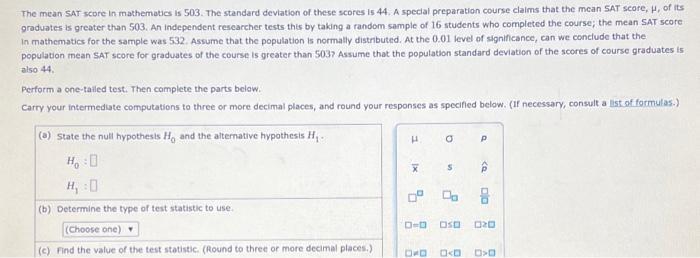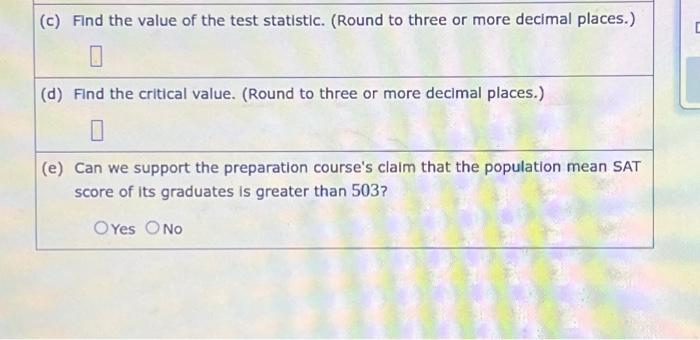Home / Expert Answers / Statistics and Probability / the-mean-sat-score-in-mathematies-is-503-the-standard-deviation-of-these-scores-is-44-a-special-pa848

# (Solved): The mean SAT score in mathematies is 503 . The standard deviation of these scores is 44. A special ...The mean SAT score in mathematies is 503 . The standard deviation of these scores is 44. A special preparation course claims that the mean SAT seore, , of its graduates is greater than 503. An independent researcher tests this by taking a random sample of 16 students who eompleted the course; the mean sat seore in mathematics for the sample was 532. Assume that the population is normally distributed. At the 0.01 level of signincance, can we conelude that the population mean SAT score for graduates of the course is greater than 503 ? Assume that the poputation standard deviation of the scores of course graduates is also 44, Perform a one-talied test. Then complete the parts below. Carry your intermediate computations to three or more decimal places, and round your responses as specified below. (if necessary, consult a list of formulas.) (a) State the null hypothesis and the alternative hypothesis . (b) Determine the type of test statistic to use. (c) Find the value of the test statistic. (Round to three or more decimal places.) (d) Find the critical value. (Round to three or more decimal places.) (e) Can we support the preparation course's claim that the population mean SAT score of its graduates is greater than 503 ? Yes No

We have an Answer from Expert